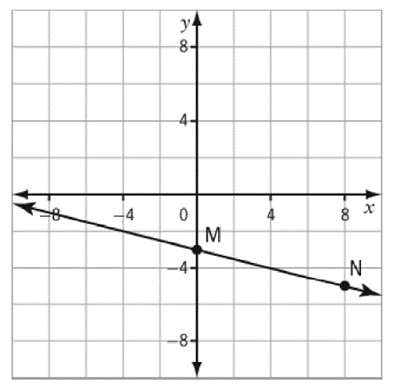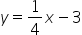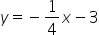Mathematics
Easy

Question

# Identify the equation that represents the line containing points M and N?## y = –4x – 3y = 4x – 3Hint:

## The correct answer is:### GIVEN-The given line passes through the points M & N as shown in the given graph.TO FIND-Equation of the given line.SOLUTION-From the given graph, we observe that-M (0,-3) & N (8,-5)We know that when a line passes through a given point, the coordinates of that point satisfies the equation of the said line.Hence, we substitute the coordiantes of M & N in the given options to find the correct equation, for which LHS = RHS.a. y = 4x - 3We substitute M (0,-3) in the given equation-    y = 4x - 3∴ -3 = 4(0) - 3∴ -3 = 0 - 3∴ -3 = -3∴ LHS = RHSWe substitute N (8,-5) in the given equation-    y = 4x - 3∴ -5 = 4(8) - 3∴ -5 = 32 - 3∴ -5 ≠ 29∴ LHS ≠ RHSSince, N (8,-5) does not satisfy the given equation, y = 4x - 3 is not the equation of the given line.b. y = 1/4 x - 3We substitute M (0,-3) in the given equation-    y = 1/4 x - 3∴ -3 = 1/4 (0) - 3∴ -3 = 0 - 3∴ -3 = -3∴ LHS = RHSWe substitute N (8,-5) in the given equation-    y = 1/4 x - 3∴ -5 = 1/4 (8) - 3∴ -5 = 2 - 3∴ -5 ≠ -1∴ LHS ≠ RHSSince, N (8,-5) does not satisfy the given equation, y = 1/4 x - 3 is not the equation of the given line.c. y = - 1/4 x - 3We substitute M (0,-3) in the given equation-    y = -1/4 x - 3∴ -3 = -1/4 (0) - 3∴ -3 = 0 - 3∴ -3 = -3∴ LHS = RHSWe substitute N (8,-5) in the given equation- y = 1/4 x - 3∴ -5 = -1/4 (8) - 3∴ -5 = -2 - 3∴ -5 = -5∴ LHS = RHSSince both M (0,-3) and N (8,-5) satisfy the given equation, y = -1/4 x - 3 is the equation of the given line.Final Answer:-Option 'c' i.e. 'y = -1/4 x - 3' is the correct answer to the given question.

Alternatively, we can use the 2 point formula to find the equation of the given line-
When we have 2 points that lie on a given line then we can find the equation of the said line by using the 2-point formula-
(y-y1) = (y2-y1) * (x-x1)
(x2-x1)
In the given question, x1 = 0, y1 = -3, x2 = 8 & y2 = -5
∴      (y-y1) = (y2-y1) * (x-x1)
(x2-x1)
∴    [y- (-3)] = [-5 - (-3)] * (x - 0)
(8-0)
∴      (y + 3) = (-5 + 3) * x
8
∴ 8 * (y + 3) = (-5 + 3) * x ................................... (Multiplying both sides by 8)
∴     8y + 24 = -2x
∴             8y = -2x - 24 ....................................... (Isolating y in LHS)
∴               y = -2/8 x - 24/8 .................................... (Dividing both sides by 8)
∴               y = -1/4 x - 3

### Related Questions to study#### With Turito Foundation.#### Get an Expert Advice From Turito.